## Newton's Second Law - Complete Toolkit

### Objectives

1. To identify the type and relative magnitude of the individual forces that act upon an object and to use such information to construct free-body diagrams for a physical situation.
2. To understand the meaning of net force and to use such an understanding to relate the values of individual forces to the value of the net force.
3. To distinguish between the concepts of mass and weight and be able to perform calculations involving mass and weight.
4. To state Newton's second law of motion, to express in equation form, and to use it to solve for acceleration, mass, or net force if knowledge of two of these three variables are known.
5. To use Newton's second law equation as a guide to thinking about the relationship between force, mass, and acceleration.
6. To use free-body diagrams and the Newton’s second law equation to determine the acceleration of an object.
7. To use free-body diagrams and the Newton’s second law equation to determine the value of an individual force that acts upon an object.

### Readings from The Physics Classroom Tutorial

1. The Physics Classroom Tutorial, Newton’s Laws Chapter, Lesson 2

http://www.physicsclassroom.com/class/newtlaws/Lesson-2/The-Meaning-of-Force

2. The Physics Classroom Tutorial, Newton’s Laws Chapter, Lesson 3

http://www.physicsclassroom.com/class/newtlaws/Lesson-3/Newton-s-Second-Law

### Physics Education Research

1. Free-Body Diagrams

Are students who use free-body diagrams more successful in interpreting physical processes and solving problems? For our most successful students, why and when do they independently construct FBD’s?

Here’s a free research article (courtesy of the American Physical Society) that delves into these exact questions.   http://journals.aps.org/prstper/abstract/10.1103/PhysRevSTPER.5.010108

Excerpt:
“We conducted a two-year quantitative and qualitative study of students' use of free-body diagrams while solving physics problems. We found that when students are in a course that consistently emphasizes the use of free-body diagrams, the majority of them do use diagrams on their own to help solve exam problems even when they receive no credit for drawing the diagrams. We also found that students who draw diagrams correctly are significantly more successful in obtaining the right answer for the problem. Lastly, we interviewed students to uncover their reasons for using free-body diagrams. We found that high achieving students used the diagrams to help solve the problems and as a tool to evaluate their work while low achieving students only used representations as aids in the problem-solving process.”

### Interactive Simulations

1.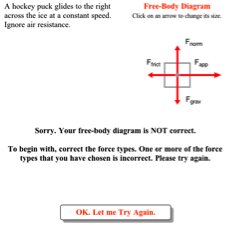Physics Interactives: Free Body Diagrams

Students are presented with 12 physical situations for which they must construct free-body diagrams. Students select a force type and adjust its magnitude so that it’s consistent with the physical situation. Feedback regarding force choices are immediate and students are given multiple opportunities to correct mistakes. This HTML5-friendly interactive has a built-in score-keeping, making it a perfect tool in a 1:1 classroom.

2.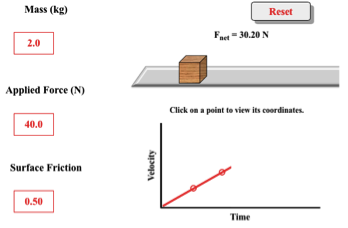Physics Interactives: Force

This interactive simulation allows students to probe the relationship between net force, mass and acceleration. A force is applied to push a box across a horizontal surface. The mass of the box, the magnitude of the force, and the amount of friction can be adjusted. A plot of velocity vs. time is provided. Clicking on the graph displays coordinates from which the slope can be calculated. The Interactive is accompanied by two different activities designed for different student abilities and different purposes. The Interactive is HTML5-friendly and does not use any plug-ins.

3.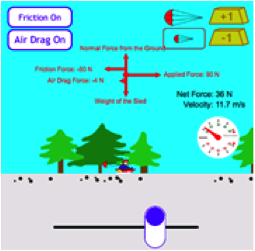Physics Interactives: Rocket Sledder

This HTML5 simulation allows users to explore the effect of balanced and unbalanced forces upon an object's motion. As a rocket-propelled sled moves across a snowy surface, the speedometer changes to demonstrate the effect of an unbalanced force upon the speed. Force diagrams are depicted during the motion. The amount of friction and air resistance and the amount of mass and thrust can all be altered.

4.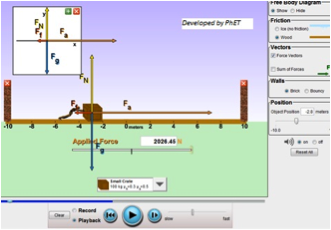PhET Simulation:  Forces and MotionThis interactive simulation provides four components for exploring balanced and unbalanced forces. Students can choose from among 5 objects of different masses, set the surface with or without friction, then "push" the object along a straight line. The simulation displays force vectors and free body diagrams to match the motion. Record your "push" and replay to see the sum of forces. The second activity focuses on the role of friction when objects are pushed on a wood surface. Set your own gravitational constant and watch the effects on static and kinetic friction. The third activity lets users display simultaneous graphs of applied force, acceleration, velocity, and position. The final activity, "Robot Moving Company", is a game where users apply a force to deliver objects of different mass from one point to another.

Editor's Note: This simulation may be adapted to a broad range of student capabilities.

5. Concord Consortium:  Spring and Mass ModelExplore forces that affect a spring’s motion in this simple model. Students can adjust spring constant, starting position, mass of the weight, and damping. Try changing one variable at a time to see how this affects the motion. You can also change the gravitational acceleration.  Why we like it:  This model provides an inquiry-based way to investigate the various forces at play in a common spring device. A large graph of Distance From Equilibrium vs. Time is run in real time as the simulation plays.

### Video and Animations

1.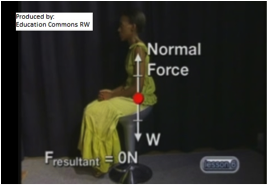Education Commons RW: Friction and the Normal Force

15-minute video could be used as a flipped lesson, for students with reading disabilities, or for learners who are struggling with the concept of forces. It investigates two of the most common contact forces: friction and the normal force. It provides multiple real-world examples, gives explicit guidance in how to identify and label both forces in free-body diagrams, and demonstrates how to apply these concepts to solve problems.

2.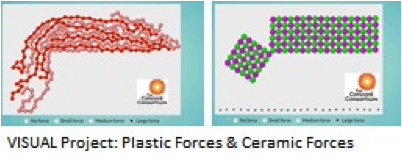Concord Consortium:  VISUAL Project

This new set of computer-based visualizations explores what happens at the molecular level when force is applied to various types of materials -- ceramics, metals, plastic, and rubber tires. The authors' purpose is to encourage learners to interpret conceptually how force interactions at the nanoscale affect an object's properties at the macroscale.  Mobile-friendly HTML5 version available.

3.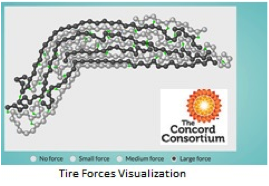Concord Consortium:  Tire Forces

We like this simple animation because it underscores the importance of molecular structure in how a material will respond to an applied force. In this case, the material is tire rubber -- a polymer composed of long molecular chains. The animation lets you choose from a "Small", "Medium", or "Large" applied force, then watch the molecular arrangement change. It also meets specific Next Generation Science Standards-Structure of Matter.  Mobile-friendly HTML5 version available.

4.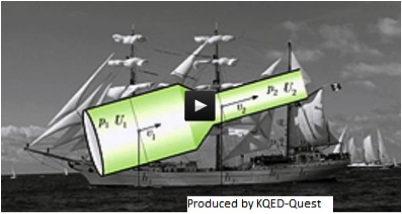QUEST: The Physics of Sailing

This high-definition 10-minute video explains how modern sailboats move forward by generating lift. The video explores the aerodynamic forces generated by two parts of the sailboat: the sails and the keel. These forces, when properly adjusted by the sailor, counteract each other to generate forward movement. Editor’s Note: Old World sailboats relied on wind to push them forward. Modern sailboats are much more complicated and offer a great opportunity to explore Bernoulli’s Principle, lift, and drag.

### Labs and Investigations

1. The Physics Classroom, The Laboratory, Wait! Hmmm. Gee.

Students use a hooked mass set and a force probe (or force scale) to investigate experimentally derive the mathematical relationship between weight and mass.

2. The Physics Classroom, The Laboratory, F-m-a Lab

Students use a motion detector and a force probe to quickly determine the mathematical relationship between force, mass and acceleration.

3. The Physics Classroom, The Laboratory, Coffee Filter Skydiver Lab

Students investigate the changes in velocity and acceleration of a falling coffee filter and relate the findings to Newton’s second law of motion.

4. The Physics Classroom, The Laboratory, Friction

Students pull a wood block across a surface. By conducting numerous trials with varying amounts of mass on the block, students determine a coefficient of static and kinetic friction from a plot of friction force vs. normal force.

5. The Physics Classroom, The Laboratory, Mu Shoe Phyzx

This is an open-ended lab project in which students evaluate the traction of a variety of shoes.

6. The Physics Classroom, The Laboratory, Normal Force-o-Meter

Students use Hooke’s law to construct a normal force-o-meter and then use the force meter to measure normal forces in a variety of situations.

### Minds On Physics Internet Modules:

The Minds On Physics Internet Modules are a collection of interactive questioning modules that target a student’s conceptual understanding. Each question is accompanied by detailed help that addresses the various components of the question.
1. Newton’s Laws, Ass’t NL4 -  Types of Forces
2. Newton’s Laws, Ass’t NL5 -  Force Diagrams
3. Newton’s Laws, Ass’t NL6 -  Mass vs. Weight
4. Newton’s Laws, Ass’t NL7 -  Newton’s Second Law
5. Newton’s Laws, Ass’t NL8 -  F = m•a Calculations
6. Newton’s Laws, Ass’t NL9 -  Force Analysis
7. Newton’s Laws, Ass’t NL10 -  Free Fall Acceleration
8. Newton’s Laws, Ass’t NL11 -  Air Resistance

### Concept Building Exercises:

1. The Curriculum Corner, Newton’s Laws, Recognizing Forces
2. The Curriculum Corner, Newton’s Laws, Mass and Weight
3. The Curriculum Corner, Newton’s Laws, Newton’s Second Law of Motion
4. The Curriculum Corner, Newton’s Laws, Net Force and Acceleration
5. The Curriculum Corner, Newton’s Laws, Free Body Diagrams
6. The Curriculum Corner, Newton’s Laws, Newton’s Second Law
7. The Curriculum Corner, Newton’s Laws, Drawing Free Body Diagrams
8. The Curriculum Corner, Newton’s Laws, Friction
9. The Curriculum Corner, Newton’s Laws, Air Resistance and Terminal Velocity
10. The Curriculum Corner, Newton’s Laws, Skydiving
11. The Curriculum Corner, Newton’s Laws, The Elephant and the Feather
12. The Curriculum Corner, Newton’s Laws, Newton’s Second Law and Problem-Solving

### Problem-Solving Exercises:

1. The Calculator Pad, Newton’s Laws of Motion, Problems #1 - #30

### Science Reasoning Activities:

1. Science Reasoning Center, Newton’s Laws, Carts, Bricks, and Bands
2. Science Reasoning Center, Newton’s Laws, Friction
3. Science Reasoning Center, Newton’s Laws, Coffee Filter Physics

### Real Life Connections:

1.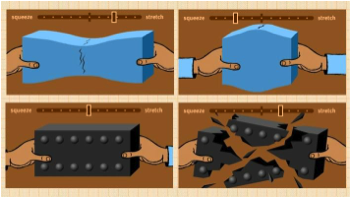PBS Learning Media:  Materials Lab

This activity simulates how eight materials with very different properties will respond to tension and compression forces. Use the slider to “stretch” or “squeeze” each material to see when it will crack or break. Materials include wood, aluminum, plastic, steel, reinforced concrete, and more. Click on “Forces” to see how external conditions such as earthquakes and temperature can affect large structures.

2.NOVA: The Physics of Stone Arches

This interactive animation explores the forces that medieval engineers considered in constructing a stone arch. To prevent collapse, they came up with some ingenious idea (like the flying buttress) to counteract the force of sideways thrust. It's a fun activity to help kids get acquainted with compression force.....could be ideal as a class opener or as a real-world example.

### Common Misconception:

1. Mass vs. Weight

Students typically struggle with the concepts of mass and weight, often confusing them with one another or even with other concepts such as volume, density and air pressure. Some students will associate the weight of an object as being the product of air resistance, air pressure or even the rotation of earth on its axis. Take some time to probe student conceptions of these two related ideas.

2. Force and the Direction of Motion

It can be predicted that students will be rather stubborn in the belief that an object that is moving to the right or upward or northward must be experiencing a force in that direction. While Newton's second law relates the direction of net force with the direction of acceleration, students will tend to relate the direction of net force with the direction of motion.

### Elsewhere on the Web:

1. Recognizing Forces

The Physics Classroom's Recognizing Forces page provides learners with 11 physical situations and the challenge of identifying the forces acting upon the object described in the situation. Learners can click on a force to identify its presence or absence and read an explanation as to why such a force is acting or not acting upon the object.  The activity serves as a great skill-building pre-cursor to drawing free-body diagrams.

### Standards:

A. Next Generation Science Standards (NGSS)

Performance Expectations
• Forces and Motion HS-PS2-1: Analyze data to support the claim that Newton’s Second Law of Motion describes the mathematical relationship among the net force on a macroscopic object, its mass, and its acceleration.
• Forces and Motion HS-PS2-6:  Communicate scientific and technical information about why the molecular-level structure is important in the functioning of designed materials.

Disciplinary Core Ideas
– High School Physical Science
• Forces and Motion - PS2.A.i: Newton’s Second Law accurately predicts changes in the motion of macroscopic objects. (Strong Alignment)
• Types of Interactions - PS2.B.iii:  Attraction and repulsion between electric charges at the atomic scale explain the structure, properties, and transformations of matter, as well as the contact forces between material objects.  (Partial alignment)

Crosscutting Concepts

Structure and Function
• High School: The functions and properties of natural and designed objects and systems can be inferred from their overall structure, the way their components are shaped and used, and the molecular substructures of its various materials.

Stability and Change
• High School: Change and rates of change can be quantified and modeled over very short or very long periods of time. Some system changes are irreversible.

Nature of Science:  Order & Consistency in Natural Systems
• High School:  Scientific knowledge is based on the assumption that natural laws operate today as they did in the past and they will continue to do so in the future.

Science and Engineering Practices

Practice #1: Analyzing and Interpreting Data
• High School:  Analyze data using tools, technologies, and/or models (e.g., computational, mathematical) in order to make valid and reliable scientific claims.  (Strong alignment)
Practice #2: Developing and Using Models
• High School:  Develop and use a model based on evidence to illustrate the relationships between systems or between components of a system.  (Strong alignment)
• High School:  Use a model to provide mechanistic accounts of phenomena.  (Strong alignment)

Practice #5: Using Mathematics and Computational Thinking
• High School:  Create or revise a computational model or simulation of a phenomenon, process, or system. (Strong alignment)
• High School:  Use mathematical representations of phenomena or design solutions to describe and/or support claims and/or explanations. (Strong alignment)

B. Common Core Standards for Mathematics – Grades 9-12

Standards for Mathematical Practice:
• Reason abstractly and quantitatively
• Model with mathematics

Quantities
• High School N-Q.1:     Use units as a way to understand problems and to guide the solution of multi-step problems; choose and interpret units consistently in formulas; choose and interpret the scale and the origin in graphs and data displays.

Functions
• High School F-IF.4     For a function that models a relationship between two quantities, interpret key features of graphs and tables in terms of the quantities, and sketch graphs showing key features given a verbal description of the relationship.
• High School F-IF.6  Calculate and interpret the average rate of change of a function (presented symbolically or as a table) over a specified interval. Estimate the rate of change from a graph.

Linear, Quadratic, and Exponential Models
• High School F-LE.1.b      Recognize situations in which one quantity changes at a constant rate per unit interval relative to another.
• High School F-LE.5      Interpret the parameters in a linear or exponential function in terms of a context.

C. Common Core Standards for English/Language Arts (ELA) – Grades 9-12

Note:  Flesch-Kincaid Reading Ease Score for text in The Physics Classroom Tutorial-Newton’s Second Law:  61.94, correlating to Grade 8.
Gunning-Fogg Index score: Grade 9

Reading Standards: Literacy in Science and Technical Subjects– Key Ideas & Details
• High School RST11-12.3     Follow precisely a complex multistep procedure when taking measurements or performing technical tasks; analyze the specific results based on explanations in the text.
• High School RST.11-12.2     Determine the central ideas or conclusions of a text; summarize complex concepts, processes, or information presented in a text by paraphrasing them in simpler but still accurate terms.

Reading Standards: Literacy in Science and Technical Subjects – Integration of Knowledge and Ideas
• High School RST.11-12.7     Integrate and evaluate multiple sources of information presented in diverse formats and media (e.g., quantitative data, video, multimedia) in order to address a question or solve a problem.
• High School RST.11-12.9    Synthesize information from a range of sources (e.g. texts, experiments, simulations) into a coherent understanding of a process, phenomenon, or concept.

Reading Standards: Literacy in Science and Technical Subjects – Range of Reading and Level of Text Complexity
• High School RST.11-12.10    By the end of Grade 10, read and comprehend science/technical texts in the Grade 9-10 text complexity band independently and proficiently.

D. College Ready Physics Standards (Heller and Stewart)

Standard 3:  Newton’s Laws of Motion – Grades 9-12

Objective 3.2:  Forces and Changes in Motion – Middle School
• M.3.2.2 – Newton’s second law of motion includes the following ideas:When multiple forces are acting on an object, the change in motion of the object is determined by the sum of the forces (Newton’s second law), which can be found using vector addition. The sum of the forces is not a real force caused by an interacting object; it is the single force that could replace the original multiple forces and cause the same change in motion.
• M.3.2.3 – The forces acting on an object can be represented by arrows (vectors) drawn on an isolated picture of the object, called a force diagram. The direction of each arrow shows the direction of the push or pull. Forces are labeled: (type of interaction) push or pull of (interacting object) on the (object of interest). For example:  “drag push of the wind on the sails of the boat”.

Objective 3.2:  Forces and Changes in Motion – High School
• H.3.2.1 – Newton’s second law of motion includes the following ideas:
1. The linear acceleration of an object is directly proportional to the vector sum of all the forces acting on the object and inversely proportional to the object’s mass (a = "F/m). The vector sum of all the forces (net force) is not a real force caused by an interaction with another object. The single force that could replace the original multiple forces and cause the same acceleration of the object is the vector sum of forces.
2. A special case of Newton’s second law occurs when the vector sum of all the forces (net force) on an object is zero. In this case, there is no acceleration and the object remains at rest or maintains a constant speed and a constant direction of motion.